# (Sample) Op-amp circuit analysis using a transfer function - Result -

This tool determine the transfer function from a inverting / non-inverting amplifier circuit. The transfer function is simulated frequency analysis and transient analysis on graphs, showing Bode diagram, Nyquist diagram, Impulse response and Step response.

### Op-amp circuit: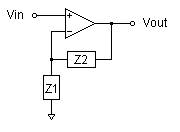Z1 Z2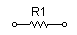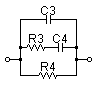#### (Sample) Transfer function from the Op-amp circuit:

 G(s)= s2+1111000s+1010000000 s2+111000s+10000000

#### Pole(s)

p = -14.349939362259[Hz]
|p|= 14.349939362259[Hz]
p = -17651.848743838[Hz]
|p|= 17651.848743838[Hz]

#### Zero(s)

z = -144.80489759888[Hz]
|z|= 144.80489759888[Hz]
z = -176676.3368775[Hz]
|z|= 176676.3368775[Hz]

#### Final value of the step response (on the condition that the system converged when t goes to infinity)

g(∞) = 101

 Z1 Z2 R1= Ω R2= Ω C1= F R3= Ω R4= Ω C3= F C4= F
p:pico, n:nano, u:micro, k:kilo, M:mega

### Select the Op-amp circuit

Inverting amplifier  Non-inverting amplifier

### Select Z1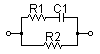### Select Z2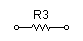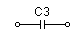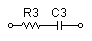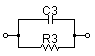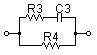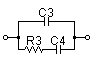### Frequency analysis

Bode diagram
Phase  Group delay
Nyquist diagram(f=0→∞)
Pole, zero
Phase margin
Oscillation analysis

Upper and lower frequency limits:
f1= - f2=[Hz] (frequency limits are optional)

### Transient analysis

Step response
Impulse response
Overshoot
Final value of the step response

Simulation time:
0〜[sec] (optional)

# Frequency analysisGain characteristics at the Bode Diagram (provides up to 1 minute)

Phase characteristics at the Bode Diagram (provides up to 1 minute)

Bode Diagram text data (provides up to 1 minute)

# Transient analysis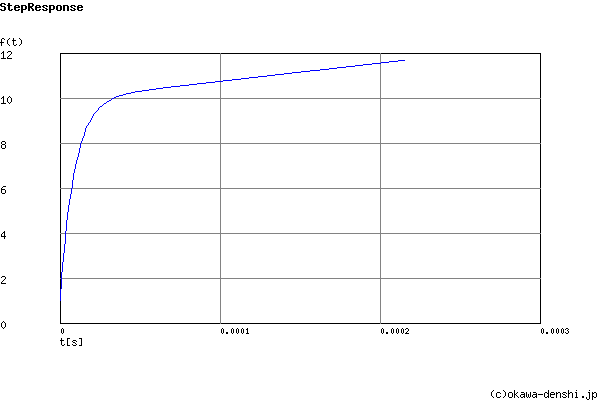Step Response text data (provides up to 1 minute)